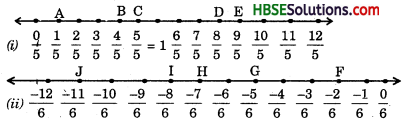# HBSE 8th Class Maths Solutions Chapter 1 Rational Numbers InText Questions

Haryana State Board HBSE 8th Class Maths Solutions Chapter 1 Rational Numbers InText Questions and Answers.

## Haryana Board 8th Class Maths Solutions Chapter 1 Rational Numbers InText Questions

Try These (Textbook Page No. 4)

Question 1.
Fill in the blanks in the following table :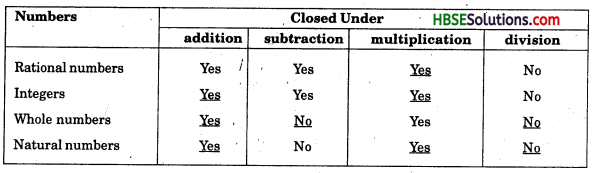Try These (Textbook Page No. 6)

Question 1.
Complete the following table :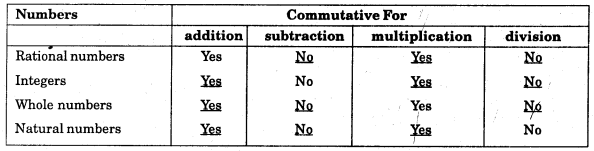Try These (Textbook Page No. 9)

Question 1.
Complete the following table :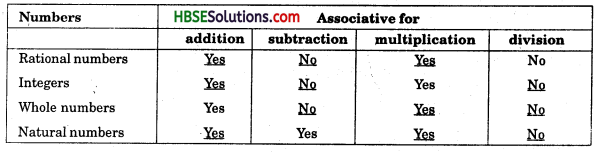Try These (Textbook Page No. 13)

Question 1.
Find using distributivity :
(i) $$\left\{\frac{7}{5} \times\left(\frac{-3}{12}\right)\right\}$$ + $$\left\{\frac{7}{5} \times \frac{5}{12}\right\}$$
(ii) $$\left\{\frac{9}{16} \times \frac{4}{12}\right\}$$ + $$\left\{\frac{9}{16} \times \frac{-3}{9}\right\}$$
Solution: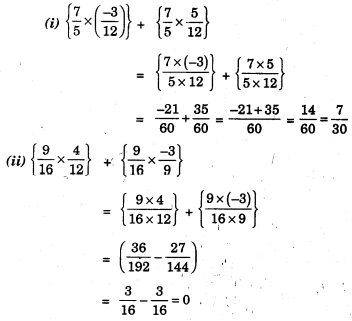Try These (Textbook Page No. 17)

Question 1.
Write the rational number for each point labelled with a letter.
(i)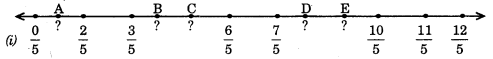(ii)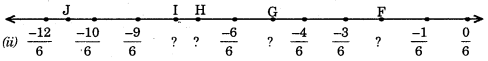Solution: